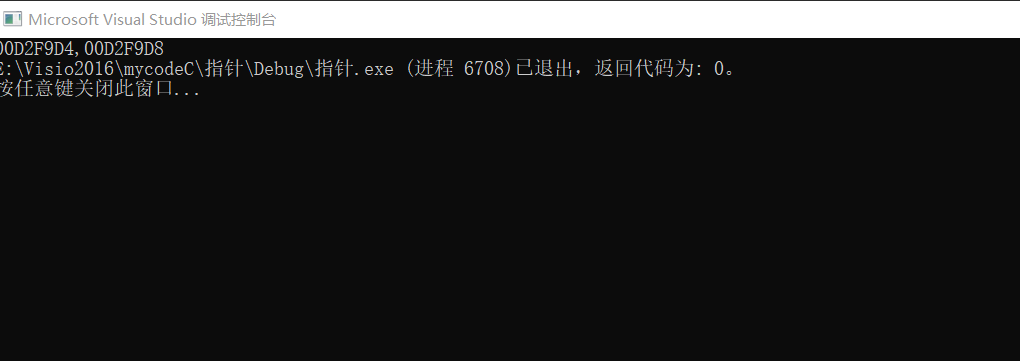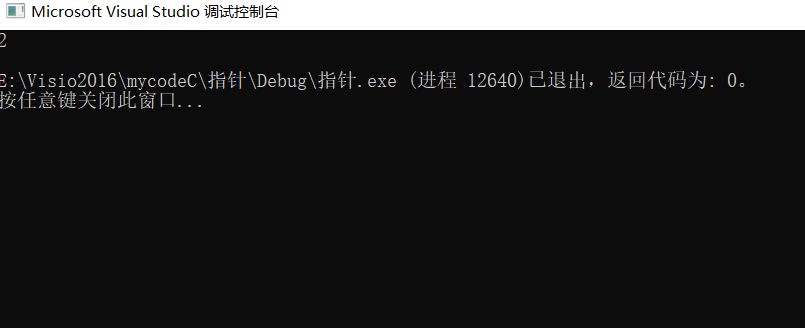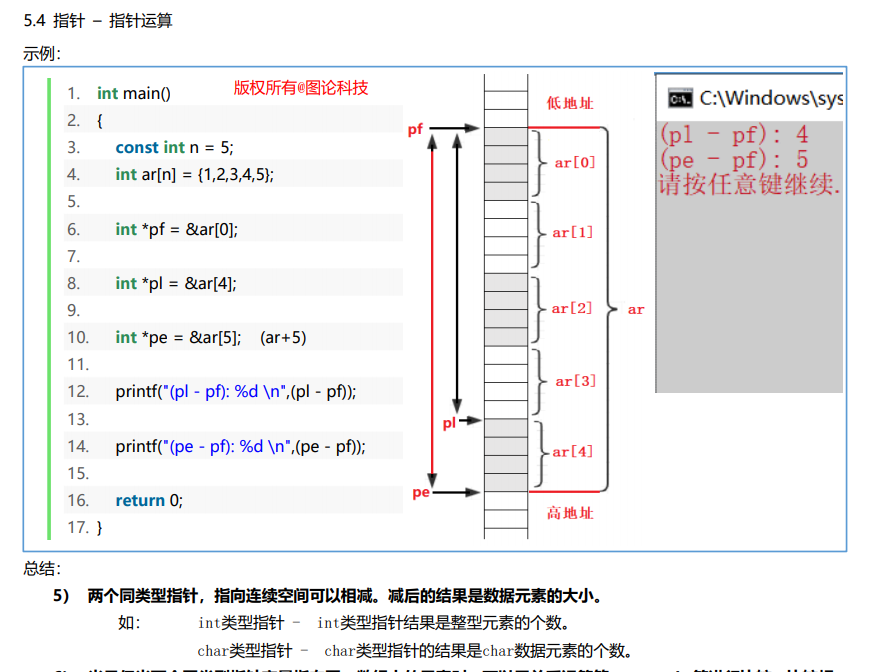• 将指向double的指针加1后，如果系统对double使用8字节存储，则数值增加8字节。 下面的例子展示了指针、数组和指针算数。指针和指针都可以通过指针和数组的方式获取数组的值。 #include <iostream> int main()...

指针、数组和指针算数

指针和数组基本等价的原因在于指针算数c++内部处理数组的方式。指针变量加1，增加的量等于它指向的类型的字节数。将指向double的指针加1后，如果系统对double使用8字节存储，则数值增加8字节。
下面的例子展示了指针、数组和指针算数。指针和指针都可以通过指针和数组的方式获取数组的值。

#include <iostream>

int main()
{
using namespace std;
double wages[] = { 10000,20000,30000 };
short stacks[] = { 3,2,1 };

double* pw = wages;
short* ps = &stacks;

cout << "pw = " << pw << ", *pw = " << *pw << endl;
pw = pw + 1;
cout << "pw + 1   " << endl;
cout << "pw = " << pw << ", *pw = " << *pw << "\n\n";

cout << "ps = " << ps << ", *ps = " << *ps << endl;
ps = ps + 1;
cout << "ps + 1   " << endl;
cout << "ps = " << ps << ", *ps = " << *ps << "\n\n";

cout << "access elements with array notation\n";
cout << "stacks= " << stacks <<", stacks= "<<stacks << "\n\n";

cout << "access elements with pointer notation\n";
cout << "*stacks= " << *stacks << ", *(stacks+1)= " << *(stacks + 1) << "\n\n";

cout << sizeof(stacks) << " size of stacks\n";
cout << sizeof(ps) << " size of ps\n\n";

ps--;
cout << "ps= " << ps << ", ps=" << ps << ", ps=" << ps << "\n";
cout << "*ps= " << *ps << ", *(ps+1)=" << *(ps+1) << ", *(ps+2)=" << *(ps+2) << "\n";

return 0;

}

输出

pw = 00DAFA28, *pw = 10000
pw + 1
pw = 00DAFA30, *pw = 20000

ps = 00DAFA18, *ps = 3
ps + 1
ps = 00DAFA1A, *ps = 2

access elements with array notation
stacks= 3, stacks= 2

access elements with pointer notation
*stacks= 3, *(stacks+1)= 2

6 size of stacks
4 size of ps

ps= 3, ps=2, ps=1
*ps= 3, *(ps+1)=2, *(ps+2)=1

c++将数组名解释为地址。多数情况下c++将数组名解释为数组第一个元素的地址。 以下2种定义方式是等价的。

double* pw = wages;
short* ps = &stacks;

从输出可知，*(stacks+1), stacks, *(ps+1), ps是等价的。通常使用数组表示法时，C++都会执行以下转换：

arrayname[i] becomes *(arrayname + 1)
如果使用的是指针，而不是数组名，c++也会执行相同的操作：
ptrname[i] becomes *(ptrname + 1)
因此，在多数情况下，可以用相同的方式使用数组名和指针名。对于他们，可以使用数组方括号表示法，也可以使用指针解除引用运算符（*）。

二者由2点不同：

1. 可以修改指针的值，而数组名是常量
ptrname = ptrname + 1;//valid
arrayname = arrayname + 1;// not valid

2. 对数组使用sizeof得到的是数组的长度，对指针使用得到的是指针的长度，即使指针指向的是一个数组。例如本例中对stacks数组和ps指针使用sizeof得到的结果：
6 size of stacks
4 size of ps

数组的地址
数组名被解释为数组第一个元素的地址，而对数组名应用地址运算符时，得到的是整个数组的地址。二者略有不同。
short tell;
cout<<tell<<endl;
cout<<&tell;

二者虽然在数值上是相同的；但是从概念上说，&tell（即tell）是一个2字节内存块的地址，而&tell是一个20字节的内存块的地址。因此，表达式tell+1将地址值加2，而&tell+1将地址值加20.

展开全文c++ 指针
• 指针存放的是地址，p=p+2，加减的数字是以指针指向的数据类型为量度的。比如 int a; int *p=a; //p初始指向a的首元素地址，p+1即指向第二个元素 int a; int *p=a; p=p+1; //p初始指向a的首行地址，p+1...

1.加减性

1. 指针存放的是地址，p=p+2，加减的数字是以指针指向的数据类型为量度的。比如
int a;
int *p=a;
//p初始指向a的首元素地址，p+1即指向第二个元素

int a;
int *p=a;
p=p+1;
//p初始指向a的首行地址，p+1指向下一行地址，
//因为这里的数据类型是int []

1. 指针之间可以相减，但不可以相加。同一类型的指针变量可以相减，表示两个指向的内存位置相隔多少个元素（注意不是字节数）

2.大小

指针间可以比较大小，但是限制同类型。表示内存中它们指向位置的高低关系

展开全文c语言
• 指针加减一个整数 指针变量的大小都是四个字节，指针加1，是加了一个指针所指向类型的大小，不是加指针变量的大小。 #include <stdio.h> int main() { int m = 0; int *p; p=&m; printf("%p%p",p,p+1);//p...

指针加减一个整数
指针变量的大小都是四个字节，指针加1，是加了一个指针所指向类型的大小，不是加指针变量的大小。

#include <stdio.h>
int main()
{
int m = 0;
int *p;
p=&m;
printf("%p%p",p,p+1);//p+1的意思是p+sizeof(*p)*1

return 0；
}指针-指针
1，指针不适合做加法运算，一般用于减法运算
2，指针做减法运算时，一定要指向同一个数组，相减结果是两个指针之间的元素的数目，而不是两个指针之间相差的字节数
3，如果两个指针不是指向同一个数组，他们相减则没有意义

#include <stdio.h>
int main() {

int a = {12,34,56,78 };
int * p,*q;
p = &a;
q = &a;
printf("%d\n",q-p);

return 0;

}展开全文c语言
• 一、几种常见的const修饰指针 1、指针所指之物，不能改变 const int *p=&a; int const*p =&a; /*以上两种写法其实都是一样的，const在*p左边，表示*p不能改变 即不能给*p再进行赋值*/ ex：//*p=100;错误 //p...

一、几种常见的const修饰指针
1、指针所指之物，不能改变

const int *p=&a;
int const*p =&a;
/*以上两种写法其实都是一样的，const在*p左边，表示*p不能改变
即不能给*p再进行赋值*/
ex：//*p=100;错误
//p=&b;正确

2、指针本身不能改变

int *const p=&a;
/*const 在指针p的坐边，则指针本身不能进行改变，即不能给p进行赋值*/
ex://p=&b;错误
//*p=100;正确

二、指针加减
1、指针加整数（1、、、）
2、指针相加没有意义
因为即使两个相同的变量连续存储，但如果相加的话，不知道会跳向哪里，指向的值很有可能是随机值，所以没有意义。
3、指针相减
遵循原则算头不算尾
int类型的指针减去int类型的指针，得到的是int类型的元素个数。同理，char、、、、展开全文• c指针加减

2021-08-24 17:18:40
指针加减（p++ & p--） 如果ptr 指向位置为 1000 的 【整数】，ptr 每增加1，它都将指向下一个【整数】位置，即当前位置往后移 4 字节，指向【 1004】 位置。 如果 ptr 指向一个地址为 1000 的【字符】，ptr 每...c语言 c++ 数据结构
• 例如： 16位编译器 char ：1个字节 char*(即指针变量): 2个字节 short int : 2个字节 int： 2个字节 unsigned int : 2个字节 float: 4个字节 double: 8个字节 long: 4个字节 long long: 8个字节 unsigned long: 4个...编译器 指针 c++ java typedef
• 指针本身也是一个变量，它存放的是某个变量的地址，它本身也是存在地址的，而指针本身也是一个变量，它所存放的是一个地址（比如0X00121212）是一个整型，所以指针变量本身是一个int类型，占据内存4个bit。...c语言
• 原理是c语言中的指针加减后，会根据指针的类型采用不同的偏移量。 比如， int* a； int* b = a+1； 则 b - a = sizeof（int） char* a； char* b = a+1; 则b - a = sizeof （char） 将上述程序改写如下，更容易...
• 许多面试官常说：C程序员新手和老手的一大差别就在于是否对指针有深刻理解，能否高效利用指针。 笔者在此建立《深入理解C指针系列》专栏与大家分享我的C指针学习。 C语言编程的精髓就是对指针的精巧使用，利用指针...c语言 c++ 后端 指针
• } 解答： 注意：1）指针加减——首先将所存储的内容转化为十六进制形式-2*4的形式-4个字节！ 然后再看指针类型-int则一次4字节，char一次1个字节 2） 十六进制中 从右至左读取是高位到低位！ 改变先从低位开始 两...
• 1、指针自增在程序中使用指针代替数组，因为变量指针可以自增，而数组不能自增，数组可以看成一个指针常量。以便顺序访问数组中的每一个元素。例如，#include const int MAX = 3;int main (){int var[] = {10, 50, ...
• 例如指针指向int，那么加减都以4个字节为单位，如果指向char，那么加减都以1个字节为单位，下面是测试代码及运行结果的截图。可见整型指针地址增加了4，字符型指针地址增加了1 2、打印字符指针的地址时，如果也直接...
• 指针变量可以加减，但是只能与整型数据加减。此时加减的含义并不是其地址值进行了加减，而是与指针指向的数据类型相关联。如果指针变量 p 的值是0x20000000，那么 p=p+2 的值并不是 0x20000002，如果在 32 位的操作...c++
• 在写文件IO时候需要用文件名和路径，发现在strncpy函数字符串相加含义的问题 char str1 = "1:/45/789/b"; //文件路径+文件名 const char *fullname = str1; //文件路径+文件名 const char *p = strrchr...c++ 字符串 指针
• 1.指针变量数据类型的作用？ 1.1指针变量在32位操作系统占用4个字节，在64位操作系统中占8个字节 指针变量占用的内存大小和指针变量的数据类型无关 1.2指针变量的数据类型是专门用于告诉操作系统，在访问指向...c语言
• 用双指针去做循环的时候，什么时候该把指针加一，何时该一，实在是令人头秃。 今天分享一个简单的小技巧——枚举法。 举个例子： 给定一个数组 nums，编写一个函数将所有 0 移动到数组的末尾，同时保持非零元素的...
• 例子1：#include int main(){float arr = {12.5, 10.0, 13.5, 90.5, 0.5};float *ptr1 = &arr; //ptr1 is 925601136float *ptr2 = ptr1 + 3;// ptr2 is 925601148printf("%f ", *ptr2);...c语言用指针进行加减
• char arr;printf("arr:\n%d\n%d\n%d\n", arr, arr + 1, arr + 2);char *parr;...从结果可以看到，字符数组每个元素占1字节，字符指针数组每个占4字节。再看一个例子：char a = 'a', b = 'b', c ...
• char arr;printf("arr:\n%d\n%d\n%d\n", arr, arr + 1, arr + 2);char *parr;...从结果可以看到，字符数组每个元素占1字节，字符指针数组每个占4字节。再看一个例子：char a = 'a', b = 'b', c ...
• 函数指针：本质上是一个指针，只不过指向函数而已 编译器在编译期间对函数开辟了一块空间，而这快空间的开始地址，就是它的函数指针 int Max(int a, int b) { return a>b?a:b; } int Add(int a, int b) { ...c语言 开发语言 数据结构
•顺序栈 栈顶 指针
• 4.3 有了智能指针，让智能指针托管这个Person对象，对象的释放就不用操心了，让智能指针管理 4.4 为了让智能指针想普通的Person指针一样使用 就要重载 -> 和 #define _CRT_SECURE_NO_WARNINGS #include<...c++
• 通过如下方式，访问指针定义范围以外的内容，指针越界 root@wesley-VirtualBox:/home/wesley/C_coding/20201130# cat pointer.c #include <stdio.h> int main() { const int a = 0x12345678; int b = 0x...
• typedef int (*fptrOperation)(int,int); int add(int num1, int num2) { return num1 + num2; } int subtract(int num1, int num2) ... return num1 - num2;...int compute(fptrOperation operation, int num1, int...
• 例如，如果您有一个使用指针的函数：def do_stuff_with_pointer(pointer, property, value): setattr(pointer, property, value)例如a_ref = ptr() # make pointera_ref.x = [1, 2] # a_ref pointer has an array...
• 加减

2021-04-25 00:00:19
类和对象-运算符重载运算符重载念：对已有的运算符重新进行定义，賦予其另一种功能·以适应不同的数据类型加减号运算符重载作用：实现两个自定义数据类型相加减的运算#include#includeusing namespace std;...
• 指针其实也是一个数据类型，它的值是一个数字，表示的是一个地址比如说：00000000 00001000 0000000000010000，对32位的计算机系统，那么指针应该也是4个字节，即有32位表示有2^32个地址，即可以遍历4g的内存空间。...
• 要搞清一个指针需要搞清指针的四方面的内容：指针的类型，指针所指向的类型，指针的值或者叫指针所指向的内存区，还有指针本身所占据的内存区。让我们分别说明。先声明几个指针放着做例子：例一：(1)int *ptr;(2)......